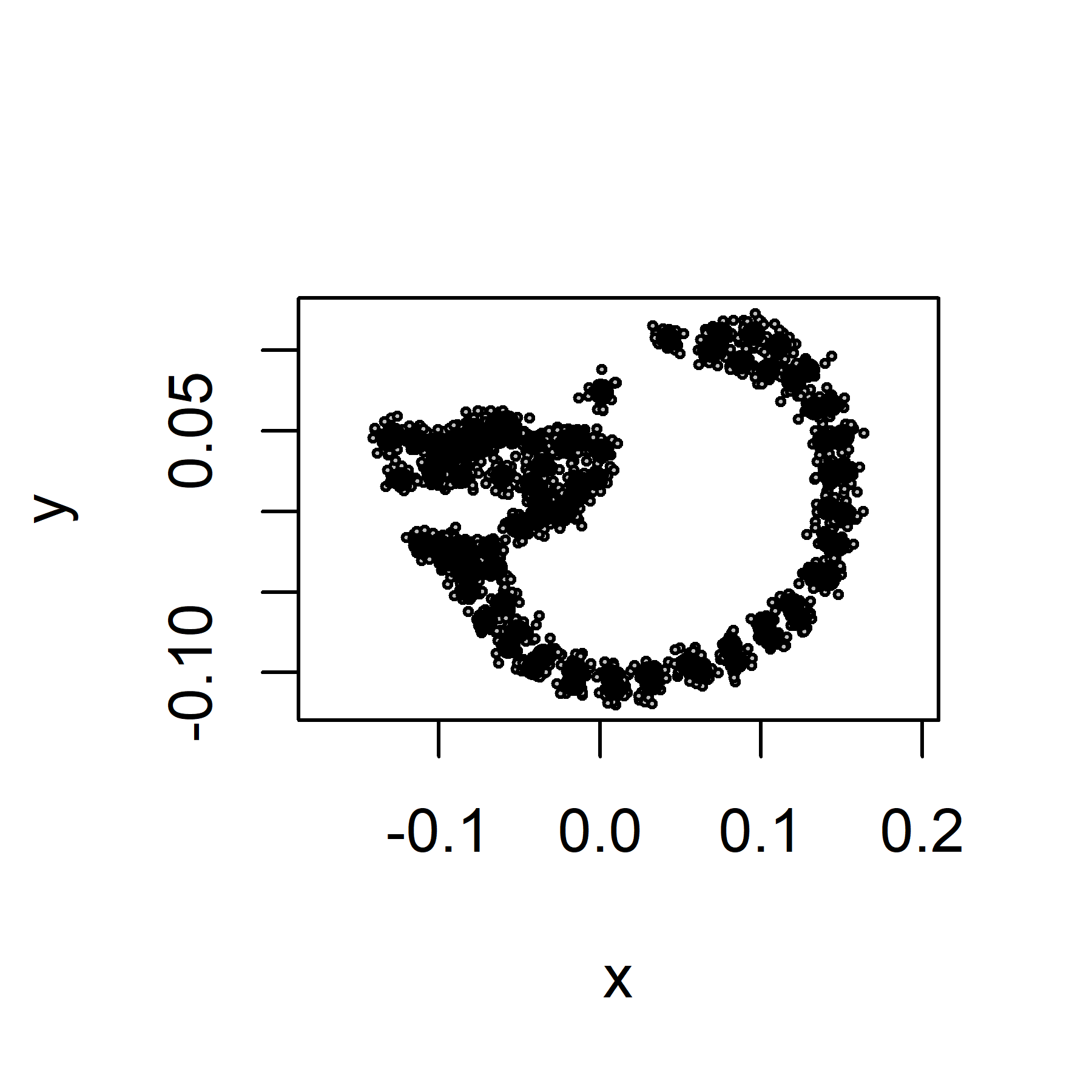# prWarp: Homo example

#### 2020-10-22

This document corresponds to the worked example of the paper Morphometric variation at different spatial scales: coordination and compensation in the emergence of organismal form. (Mitteroecker et al., 2020a). The dataset is a set of 87 2D landmarks on the midsagittal plane of the skull in 24 adult modern humans, which are also accessible via the DRYAD repository (Mitteroecker et al., 2020b). The example below describes how to decompose shape variation into partial warps, in order to obtain bending energies, principal warps, partial warp scores, and the non-affine component of shape variation for 2D landmark configurations. In also describes how to compute Mardia-Dryden distributions and self-similar distributions of landmarks. For further details about results interpretation, please read the associated paper.

## Data preparation

Install and load the packagein R.

library("prWarp")

Load the dataset HomoMidSag. This dataset comprises the coordinates of 87 two-dimensional landmarks for the 24 specimens as a data frame. Semilandmarks are already slid, but not superimposed.

data("HomoMidSag")
k <- dim(HomoMidSag) / 2  # number of landmarks
n_spec <- dim(HomoMidSag)  # number of specimens
homo_ar <- geomorph::arrayspecs(HomoMidSag, k, 2)  # create an array
## Registered S3 method overwritten by 'RRPP':
##   method         from
##   print.classify Morpho
dimnames(homo_ar)[] <- 1:k
dimnames(homo_ar)[] <- c("X", "Y")

Superimpose landmarks using the generalized Procrustes analysis (GPA).

Visualization of the mean shape

plot(m_mshape, asp = 1, main = "Average shape", xlab = "X", ylab = "Y")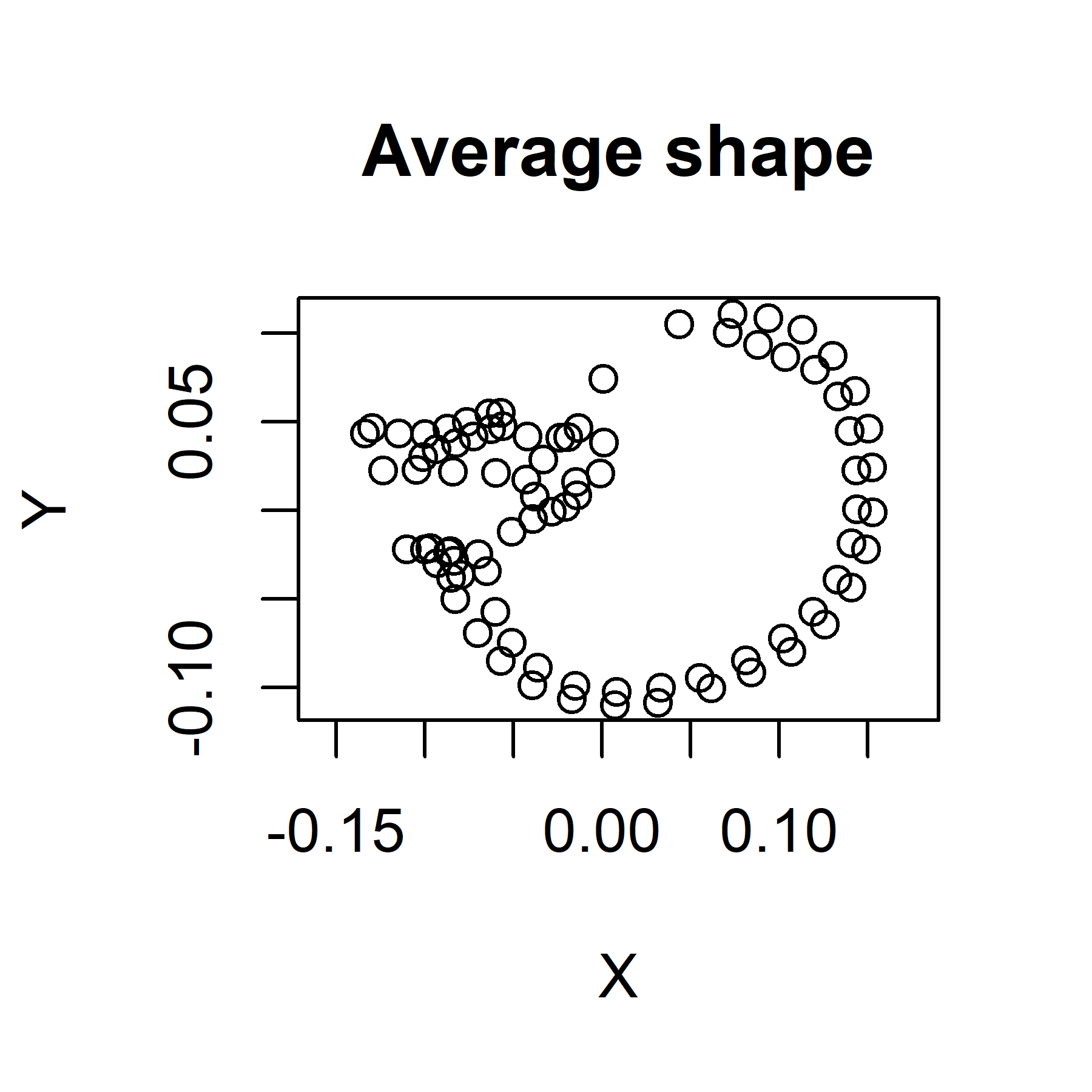## Partial warp decomposition

Decompose the 87 Procrustes aligned landmarks into partial warps. The reference matrix is generally the average landmark configuration. Note that the function create.pw.be returns principal warps, partial warp scores, partial warp variation and associated bending energies, but also the non-affine componentn of shape variation.

homo_be_pw <- create.pw.be(m_overall, m_mshape)

Plot the partial warp variance as a function of the log of the inverse bending energy. The first pairs of partial warps correspond to small-scale shape variation whereas the last pairs correspond to the large-scale shape variation.

# Computation of log BE^-1 for the (k-3) partial warps
logInvBE <- log((homo_be_pw$bendingEnergy)^(-1)) # Computation of log PW variance for the (k-3) partial warps logPWvar <- log(homo_be_pw$variancePW)
# Linear regression of the log PW variance on the log BE^-1
# Plot log PW variance on log BE^-1 with regression line
plot(logInvBE, logPWvar, col = "white", asp = 1, main = "PW variance against inverse BE", sub = paste("slope =", round(mod$coefficients, 2)), xlab = "log 1/BE", ylab = "log PW variance") text(logInvBE, logPWvar, labels = names(logPWvar), cex = 0.5) abline(mod, col = "blue")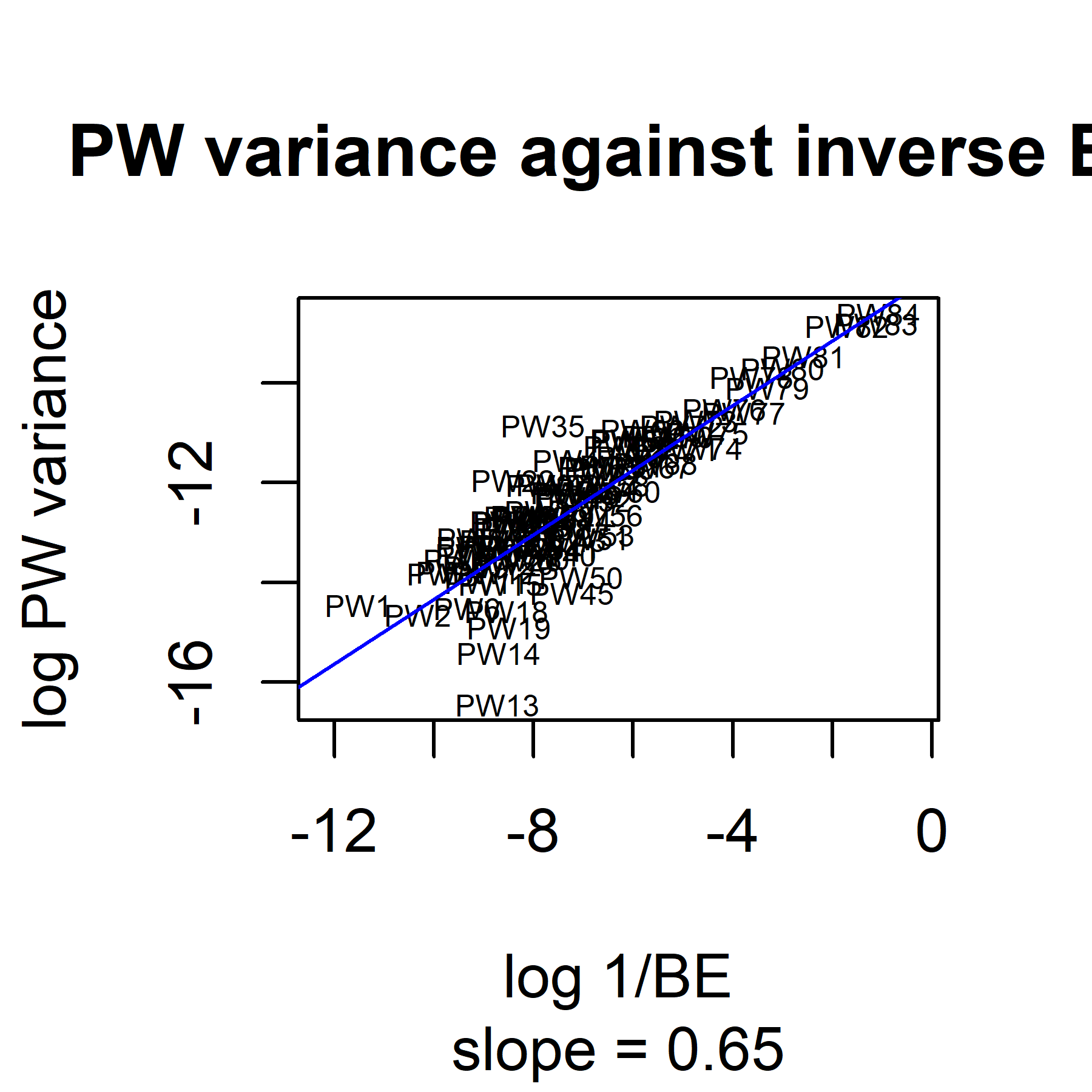Visualize the non-affine component of shape variation. # Compute the trace of t(Xnonaf) %*% Xnonaf tr_nonaf <- sum(diag(t(homo_be_pw$Xnonaf) %*% homo_be_pw$Xnonaf)) # Convert matrix into a 3D array Anonaf <- xxyy.to.array(homo_be_pw$Xnonaf, p = k, k = 2)
# Plot the non-affine shape variation around the mean
geomorph::plotAllSpecimens(Anonaf, plot.param = list(pt.cex = 0.3, mean.cex = 0.8, mean.col = "red"))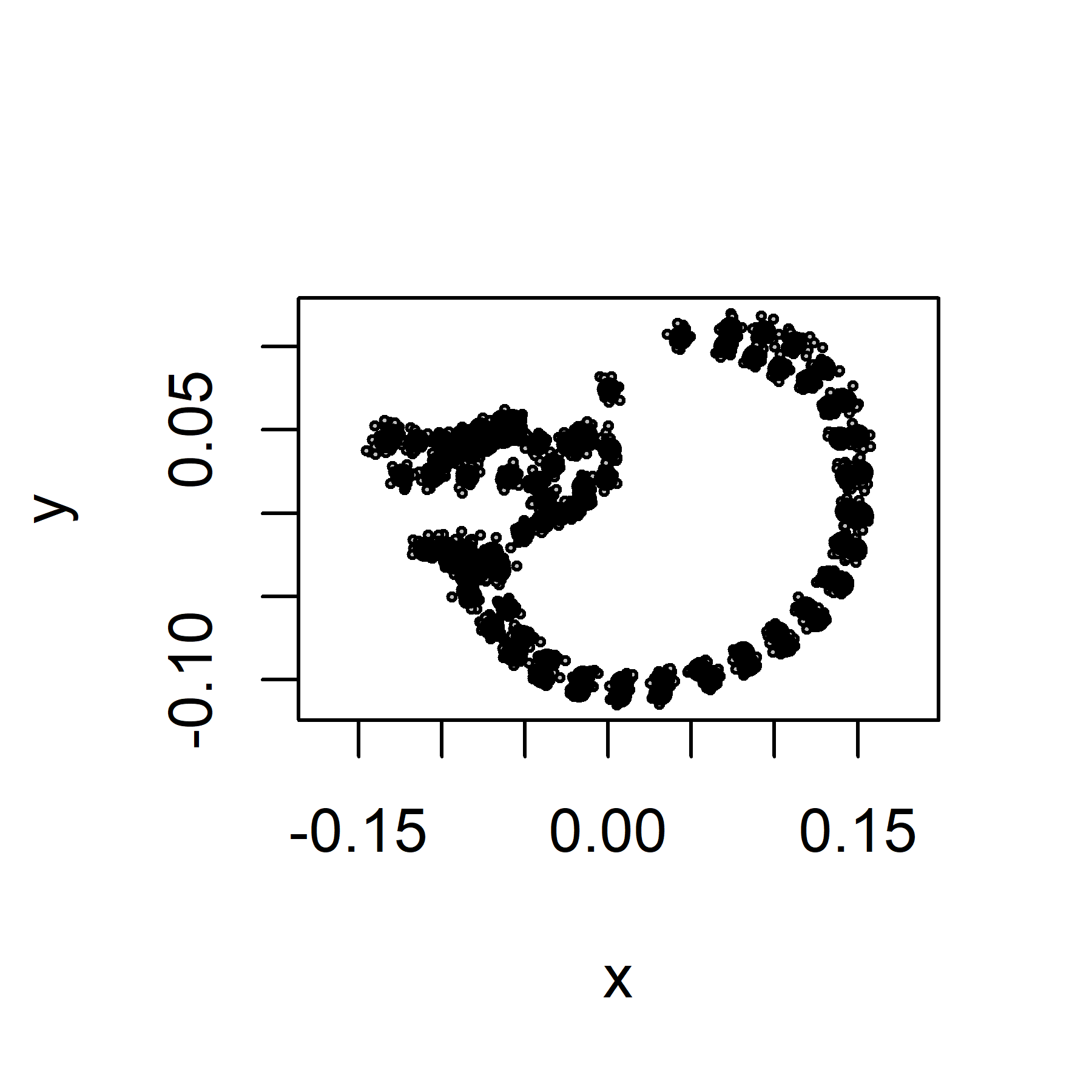## Self-similar and Mardia-Dryden distributions

Compute a self-similar distribution around the average landamark configuration.

# Compute the self-similar distribution
Xdefl <- ssim.distri(m_mshape, n = n_spec, sd = 0.05, f = 1)
# Compute the trace of t(Xdefl) %*% Xdefl
tr_defl <- sum(diag(t(Xdefl) %*% Xdefl))
# Convert matrix into a 3D array
Adefl <- xxyy.to.array(Xdefl, p = k, k = 2)
# Plot the self-similar distribution
geomorph::plotAllSpecimens(Adefl, plot.param = list(pt.cex = 0.3, mean.cex = 0.8, mean.col = "red"))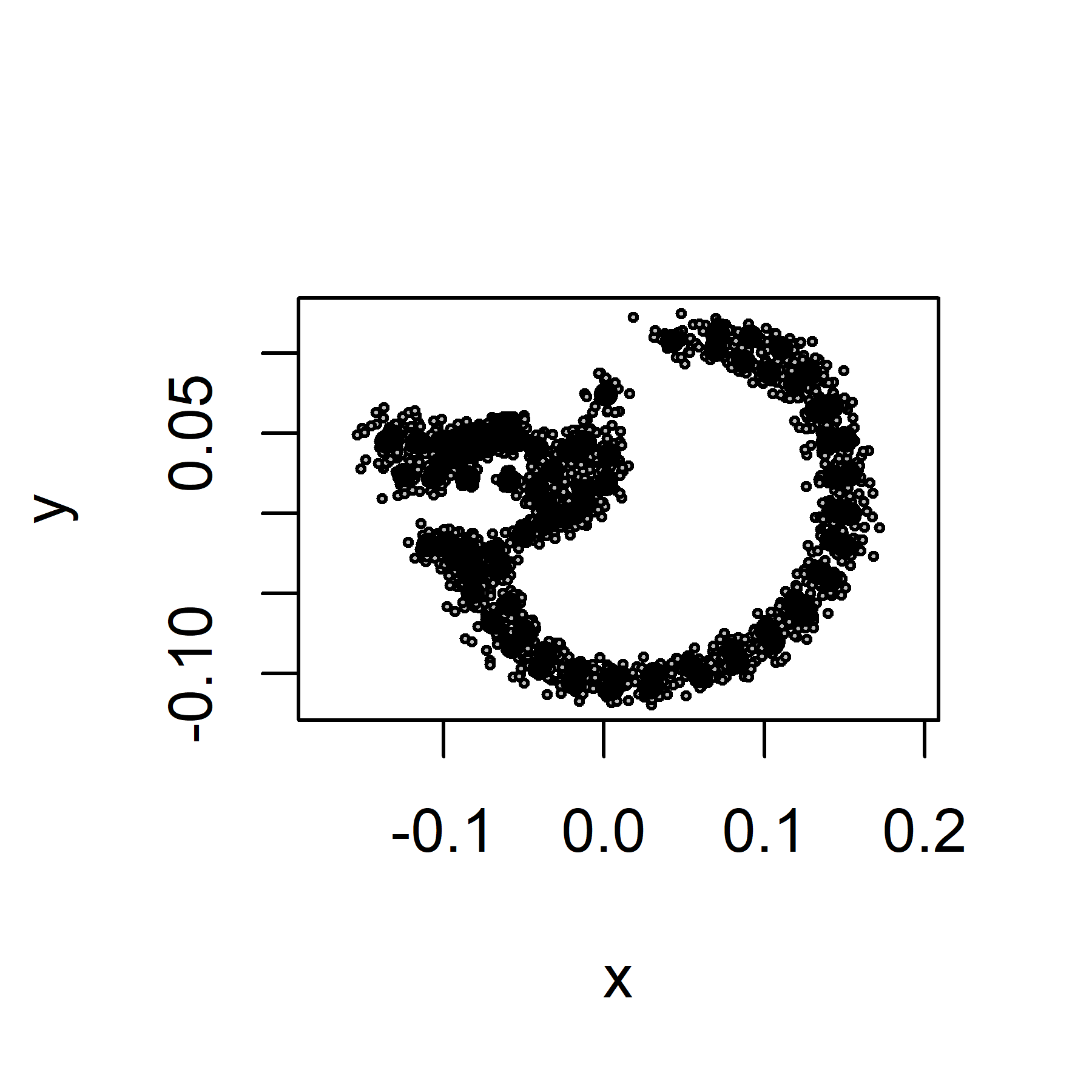Compute a Mardia-Dryden distribution around the average landamark configuration.

# Compute the Mardia-Dryden distribution
Xmd <- md.distri(m_mshape, n = n_spec, sd = 0.005)
# Convert matrix into a 3D array
Amd <- xxyy.to.array(Xmd, p = k, k = 2)
# Plot the Mardia-Dryden distribution
geomorph::plotAllSpecimens(Amd, plot.param = list(pt.cex = 0.3, mean.cex = 0.8, mean.col = "red"))